### Home > CC4 > Chapter 10 > Lesson 10.2.2 > Problem10-96

10-96.
1. Solve the equations, if possible. Be sure to find all possible solutions. Explain or justify your steps for part (a). Homework Help ✎

1. 9(x − 4) = 81

2.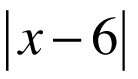= 2

3.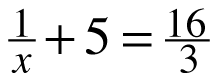4. 2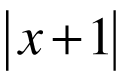= −4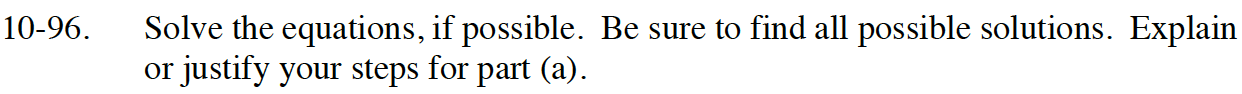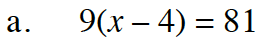Divide both sides by 9 or distribute 9 into the (x −4) factor.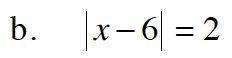The absolute value of what two numbers equals 2?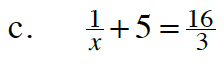Subtract 5 from both sides.

$\frac{1}{x}=\frac{1}{3} \text{ So what does }x \text{ have to be?}$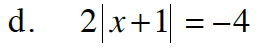Can the absolute value of a number be negative?